# Integration in Proton NMR

There is additional information obtained from 1H NMR spectroscopy that is not typically available from 13C NMR spectroscopy. Chemical shift can show how many different types of hydrogens are found in a molecule; integration reveals the number of hydrogens of each type. An integrator trace (or integration trace) can be used to find the ratio of the numbers of hydrogen atoms in different environments in an organic compound.

An integrator trace is a computer generated line which is superimposed on a proton NMR spectra. In the diagram, the integrator trace is shown in red.An integrator trace measures the relative areas under the various peaks in the spectrum. When the integrator trace crosses a peak or group of peaks, it gains height. The height gained is proportional to the area under the peak or group of peaks. You measure the height gained at each peak or group of peaks by measuring the distances shown in green in the diagram above - and then find their ratio.

For example, if the heights were 0.7 cm, 1.4 cm and 2.1 cm, the ratio of the peak areas would be 1:2:3. That in turn shows that the ratio of the hydrogen atoms in the three different environments is 1:2:3.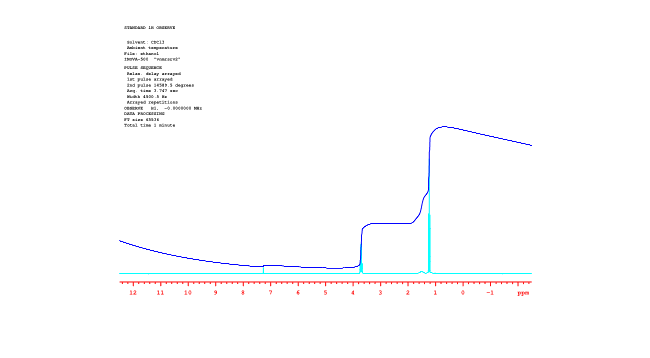Figure NMR16.1H NMR spectrum of ethanol with solid integral line. Source: Spectrum taken in CDCl3 on a Varian Gemini 2000 Spectrometer with 300 MHz Oxford magnet.

Looking at the spectrum of ethanol, you can see that there are three different kinds of hydrogens in the molecule. You can also see by integration that there are three hydrogens of one type, two of the second type, and one of the third type -- corresponding to the CH3 or methyl group, the CH2 or methylene group and the OH or hydroxyl group. That information helps narrow down the number of possible structures of the sample, and so it makes structure elucidation of an unknown sample much easier.

• integration reveals the ratio of one type of hydrogen to another within a molecule.

Integral data can be given in different forms. You should be aware of all of them. In raw form, an integral is a horizontal line running across the spectrum from left to right. Where the line crosses the frequency of a peak, the area of the peak is measured. This measurement is shown as a jump or step upward in the integral line; the vertical distance that the line rises is proportional to the area of the peak. The area is related to the amount of radio waves absorbed at that frequency, and the amount of radio waves absorbed is proportional to the number of hydrogen atoms absorbing the radio waves.

Sometimes, the integral line is cut into separate integrals for each peak so that they can be compared to each other more easily.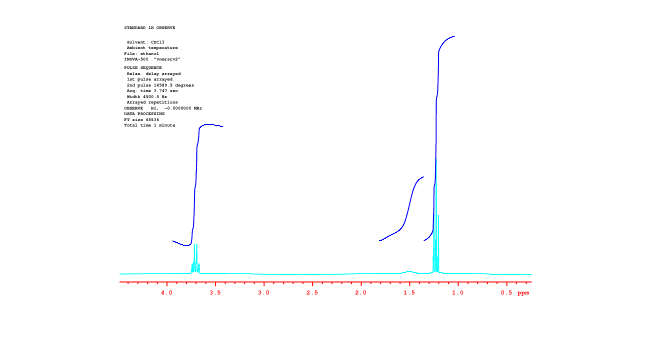Figure NMR17.1H NMR spectrum of ethanol with broken integral line. Source: Spectrum taken in CDCl3 on a Varian Gemini 2000 Spectrometer with 300 MHz Oxford magnet.

Often, instead of displaying raw data, the integrals are measured and their heights are displayed on the spectrum.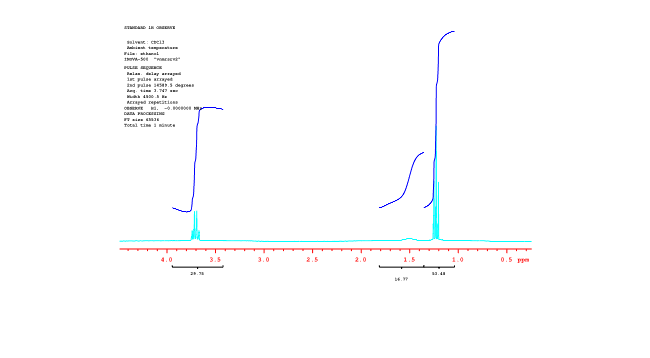Figure NMR18.1H NMR spectrum of ethanol with numerical integrals.

Source: Spectrum taken in CDCl3 on a Varian Gemini 2000 Spectrometer with 300 MHz Oxford magnet.

Sometimes the heights are "normalized". They are reduced to a lowest common factor so that their ratios are easier to compare. These numbers could correspond to numbers of hydrogens, or simply to their lowest common factors. Two peaks in a ratio of 1H:2H could correspond to one and two hydrogens, or they could correspond to two and four hydrogens, etc.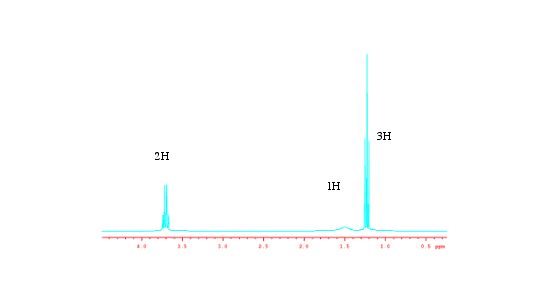Figure NMR19.1H NMR spectrum of ethanol with normalized integral numbers.

Source: Spectrum taken in CDCl3 on a Varian Gemini 2000 Spectrometer with 300 MHz Oxford magnet.

### Problem NMR.6.

Sketch a predicted NMR spectrum for each of the following compounds, with an integral line over each peak.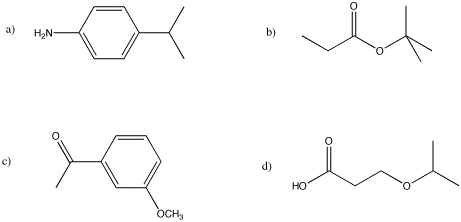### Problem NMR.7.

Measure the integrals in the following compounds. Given the integral ratios and chemical shifts, can you match each peak to a set of protons?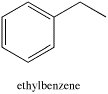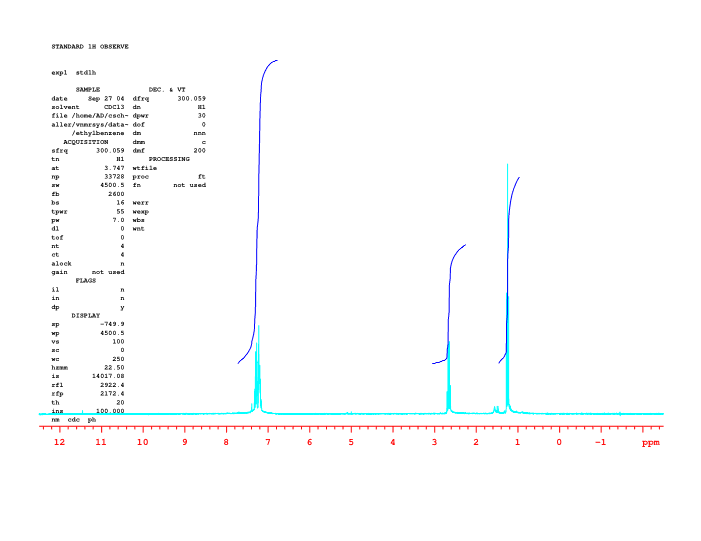## Contributors

Jim Clark (Chemguide.co.uk)